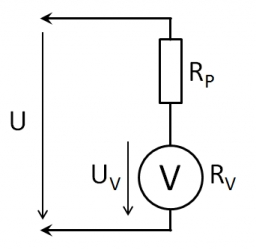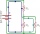# Voltmeter range

We have a voltmeter which in the original set measures voltage to 10V. Calculate the size of the ballast resistor for this voltmeter, if we want to measure the voltage up to 50V. Voltmeter's internal resistance is 2 kiloohm/Volt.

Result

R2 =  80000 Ω

#### Solution:

$U_{ 1 } = 10 \ V \ \\ U_{ 2 } = 50 \ V \ \\ \ \\ R_{ 1 } = 2 \cdot \ 1000 \cdot \ U_{ 1 } = 2 \cdot \ 1000 \cdot \ 10 = 20000 \ Ω \ \\ U_{ 3 } = U_{ 2 }-U_{ 1 } = 50-10 = 40 \ V \ \\ \ \\ I = \dfrac{ U_{ 1 } }{ R_{ 1 } } = \dfrac{ 10 }{ 20000 } = \dfrac{ 1 }{ 2000 } = 0.0005 \ A \ \\ U_{ 3 } = I \cdot \ R_{ 2 } \ \\ \ \\ R_{ 2 } = U_{ 3 }/I = 40/0.0005 = 80000 = 80000 \ \text { Ω }$Our examples were largely sent or created by pupils and students themselves. Therefore, we would be pleased if you could send us any errors you found, spelling mistakes, or rephasing the example. Thank you!

Leave us a comment of this math problem and its solution (i.e. if it is still somewhat unclear...):Be the first to comment!## Next similar math problems:

1. Effective and mean voltageA voltage divider consisting of resistors R1 = 103000 Ω and R2 = 197000 Ω is connected to the ideal sine wave voltage source, R2 is connected to a voltmeter which measures the mean voltage and has an internal resistance R3 = 200300 Ω, the measured value is
2. ConfectioneryThe village markets have 5 kinds of sweets, one weighs 31 grams. How many different ways a customer can buy 1.519 kg sweets.
3. GP membersThe geometric sequence has 10 members. The last two members are 2 and -1. Which member is -1/16?
4. Theorem proveWe want to prove the sentence: If the natural number n is divisible by six, then n is divisible by three. From what assumption we started?
5. Holidays - on poolChildren's tickets to the swimming pool stands x € for an adult is € 2 more expensive. There was m children in the swimming pool and adults three times less. How many euros make treasurer for pool entry?
6. Expression with powersIf x-1/x=5, find the value of x4+1/x4
7. CoefficientDetermine the coefficient of this sequence: 7.2; 2.4; 0.8
8. AlgebraX+y=5, find xy (find the product of x and y if x+y = 5)
9. AP - simpleDetermine the first nine elements of sequence if a10 = -1 and d = 4
10. LineIt is true that the lines that do not intersect are parallel?
11. Third memberDetermine the third member of the AP if a4=93, d=7.5.
12. SequenceWrite the first 6 members of these sequence: a1 = 5 a2 = 7 an+2 = an+1 +2 an
13. PIN - codesHow many five-digit PIN - code can we create using the even numbers?
14. BlocksThere are 9 interactive basic building blocks of an organization. How many two-blocks combinations are there?
15. AverageIf the average(arithmetic mean) of three numbers x,y,z is 50. What is the average of there numbers (3x +10), (3y +10), (3z+10) ?
16. Elimination methodSolve system of linear equations by elimination method: 5/2x + 3/5y= 4/15 1/2x + 2/5y= 2/15
17. TrigonometryIs true equality? ?